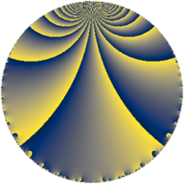# Properties

 Label 152.4.pLevel $152$ Weight $4$ Character orbit 152.p Rep. character $\chi_{152}(45,\cdot)$ Character field $\Q(\zeta_{6})$ Dimension $116$ Newform subspaces $1$ Sturm bound $80$ Trace bound $0$

# Related objects

## Defining parameters

 Level: $$N$$ $$=$$ $$152 = 2^{3} \cdot 19$$ Weight: $$k$$ $$=$$ $$4$$ Character orbit: $$[\chi]$$ $$=$$ 152.p (of order $$6$$ and degree $$2$$) Character conductor: $$\operatorname{cond}(\chi)$$ $$=$$ $$152$$ Character field: $$\Q(\zeta_{6})$$ Newform subspaces: $$1$$ Sturm bound: $$80$$ Trace bound: $$0$$

## Dimensions

The following table gives the dimensions of various subspaces of $$M_{4}(152, [\chi])$$.

Total New Old
Modular forms 124 124 0
Cusp forms 116 116 0
Eisenstein series 8 8 0

## Trace form

 $$116q - q^{2} - 7q^{4} - 11q^{6} - 8q^{7} - 46q^{8} + 484q^{9} + O(q^{10})$$ $$116q - q^{2} - 7q^{4} - 11q^{6} - 8q^{7} - 46q^{8} + 484q^{9} - 44q^{10} - 58q^{12} + 24q^{14} - 230q^{15} - 67q^{16} - 2q^{17} + 196q^{18} - 840q^{20} + 137q^{22} - 2q^{23} + 77q^{24} + 1248q^{25} + 492q^{26} - 96q^{28} - 904q^{30} + 208q^{31} - 431q^{32} - 180q^{33} + 224q^{34} - 84q^{36} + 1552q^{38} - 116q^{39} - 58q^{40} - 22q^{41} - 568q^{42} - 89q^{44} - 1852q^{46} + 202q^{47} - 89q^{48} + 5220q^{49} - 942q^{50} + 232q^{52} - 231q^{54} + 248q^{55} - 2296q^{56} - 398q^{57} - 3620q^{58} - 1378q^{60} + 614q^{62} - 796q^{63} + 1550q^{64} - 508q^{65} - 797q^{66} + 1860q^{68} - 2968q^{70} + 1986q^{71} - 1596q^{72} - 218q^{73} + 2490q^{74} - 4697q^{76} + 1254q^{78} + 1250q^{79} + 3136q^{80} - 3810q^{81} - 169q^{82} + 4136q^{84} - 2360q^{86} - 1404q^{87} + 4434q^{88} - 2q^{89} + 1378q^{90} - 1958q^{92} - 4608q^{94} + 438q^{95} + 3410q^{96} - 1586q^{97} + 55q^{98} + O(q^{100})$$

## Decomposition of $$S_{4}^{\mathrm{new}}(152, [\chi])$$ into newform subspaces

Label Dim. $$A$$ Field CM Traces $q$-expansion
$$a_2$$ $$a_3$$ $$a_5$$ $$a_7$$
152.4.p.a $$116$$ $$8.968$$ None $$-1$$ $$0$$ $$0$$ $$-8$$